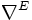# Dirac structure

(diff) ← Older revision | Latest revision (diff) | Newer revision → (diff)
A Dirac structure on a Riemannian manifold$(M,g)$ is a quadruple$(E,c,\nabla^E,\nabla^M)$ where:
•$E$ is a Hermitian vector bundle on$M$
•$c: T^*(M) \to End(E)$ is a self-adjoint Clifford structure on$E$
•$\nabla^M$ is a metric connection on$M$
•$\nabla^E$ is a Hermitian connection on$E$ compatible with Clifford multiplication
A geometric Dirac structure is a Dirac structure where the metric connection$\nabla^M$ is the Levi-Civita connection.